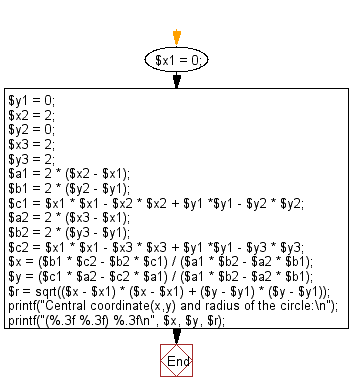﻿ PHP Exercise: Compute the radius and the central coordinate of a circle - w3resource# PHP Exercises: Compute the radius and the central coordinate of a circle

## PHP: Exercise-53 with Solution

Write a PHP program to compute the radius and the central coordinate (x, y) of a circle which is constructed by three given points on the plane surface.

Sample Solution: -

PHP Code:

``````<?php
\$x1 = 0; \$y1 = 0; \$x2 = 2; \$y2 = 0; \$x3 = 2; \$y3 = 2;
\$a1 = 2 * (\$x2 - \$x1);
\$b1 = 2 * (\$y2 - \$y1);
\$c1 = \$x1 * \$x1 - \$x2 * \$x2 + \$y1 *\$y1 - \$y2 * \$y2;
\$a2 = 2 * (\$x3 - \$x1);
\$b2 = 2 * (\$y3 - \$y1);
\$c2 = \$x1 * \$x1 - \$x3 * \$x3 + \$y1 *\$y1 - \$y3 * \$y3;
\$x = (\$b1 * \$c2 - \$b2 * \$c1) / (\$a1 * \$b2 - \$a2 * \$b1);
\$y = (\$c1 * \$a2 - \$c2 * \$a1) / (\$a1 * \$b2 - \$a2 * \$b1);
\$r = sqrt((\$x - \$x1) * (\$x - \$x1) + (\$y - \$y1) * (\$y - \$y1));
printf("Central coordinate(x,y) and radius of the circle:\n");
printf("(%.3f %.3f) %.3f\n", \$x, \$y, \$r);
?>
```
```

Sample Output:

```Central coordinate(x,y) and radius of the circle:
(1.000 1.000) 1.414
```

Flowchart:PHP Code Editor:

Have another way to solve this solution? Contribute your code (and comments) through Disqus.

What is the difficulty level of this exercise?

Test your Programming skills with w3resource's quiz.

﻿

## PHP: Tips of the Day

Why shouldn't I use mysql_* functions in PHP?

The MySQL extension:

• Is not under active development
• Is officially deprecated as of PHP 5.5 (released June 2013).
• Has been removed entirely as of PHP 7.0 (released December 2015)
• This means that as of 31 Dec 2018 it does not exist in any supported version of PHP. If you are using a version of PHP which supports it, you are using a version which doesn't get security problems fixed.
• Lacks an OO interface
• Doesn't support:
• Non-blocking, asynchronous queries
• Prepared statements or parameterized queries
• Stored procedures
• Multiple Statements
• Transactions
• The "new" password authentication method (on by default in MySQL 5.6; required in 5.7)
• Any of the new functionality in MySQL 5.1 or later

Since it is deprecated, using it makes your code less future proof.

Lack of support for prepared statements is particularly important as they provide a clearer, less error-prone method of escaping and quoting external data than manually escaping it with a separate function call.

Ref : https://bit.ly/2BIIsF0